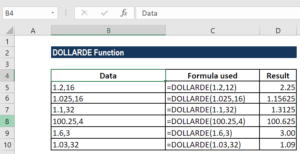# DOLLARDE Function

Converts a dollar value in fractional notation into a dollar value expressed in decimal notation

## Using DOLLARDE for US Treasury Bonds Quotes

The DOLLARDE Function is an Excel Financial function. It helps in converting a dollar value in fractional notation into a dollar value expressed in decimal notation. DOLLARDE will divide the fraction part of the value by an integer specified by the user. It is used in pricing US treasury bonds quotes, which are priced to the nearest 1/32 of a dollar. Learn more from the New York Fed.

The function is very useful for financial analysts when doing financial reports using stock market quotes, as fractional dollars are used for security prices. DOLLARDE is available from MS Excel 2007.

### Formula

=DOLLARDE(fractional_dollar, fraction)

The DOLLARDE function uses the following arguments:

1. Fractional_dollar (required argument) – This is the number expressed as an integer part and a fraction part, separated by a decimal point.
2. Fraction (required argument) – This is the integer to be used in the denominator of a fraction. We need to provide it as an integer, but in case of a decimal value, Excel will truncate it to an integer.

The function will divide the fraction part of the value by an integer. For example, if we want a security’s price to be expressed to a precision of 1/16 of a dollar, we divide the fraction part by 16. In this case, 1.2 represents \$2.25 (\$1 + 2/16 = \$2.25).

### How to use DOLLARDE Function in Excel?

To understand the uses of the DOLLARDE function, let’s consider a few examples:

#### Example 1

Let’s see how the function works using the different examples shown below:

DataFormula usedDescription
1.2,16=DOLLARDE(1.2,16)Converts the decimal number 1.2, read as 1 and 20/16. As the fraction value is 16, the price has a precision of 1/16 of a dollar.
1.025,16=DOLLARDE(1.025,16)Converts the decimal number 1.025, read as 1 and 2.5/16. As the fraction value is 16, the price has a precision of 1/16 of a dollar.
1.1,32=DOLLARDE(1.1,32)Converts 1.1, read as 1 and 10/32, to a decimal number (1.3125). As the fraction value is 32, the price has a precision of 1/32 of a dollar.
100.25,4=DOLLARDE(100.25,4)Converts 100.25, read as 100 and 1/4, to a decimal number (100.625). As the fraction value is 4, the price has a precision of 1/4 of a dollar.
1.6,3=DOLLARDE(1.6,3)Converts 1.6, read as 1 and 2/3, to a decimal number. As the fraction value is 3, the price has a precision of 1/3 of a dollar.
1.03,32=DOLLARDE(1.03,32)Converts 1.03, read as 1 and 1/32, to a decimal number. As the fraction value is 32, the price has a precision of 1/32 of a dollar.

We get the results below:### A few notes about the DOLLARDE Function

1. If the fraction given is not an integer, it will be truncated.
2. #NUM! error – Occurs when the fraction is less than zero.
3. #DIV/0 error – Occurs when the fraction given is zero.
4. #VALUE! error – Occurs if any of the given arguments is non-numeric.
5. The DOLLARDE function is useful for converting values that are given as 16¼ to the decimal and using them in other calculations or charts.
6. The function accepts a reference to a cell, so we can provide the arguments in a different cell and refer to them in the DOLLARDE formula.
7. For users using MS Excel 2003, remember that the function is only available when the Analysis ToolPak add-in is loaded.

Thanks for reading CFI’s guide to important Excel functions and how DOLLARDE can help with pricing US treasury bonds quotes, to the closest 1/32 of a dollar. By taking the time to learn and master these functions, you’ll significantly speed up your financial analysis. To learn more, check out these CFI additional resources:

• Excel Functions for Finance